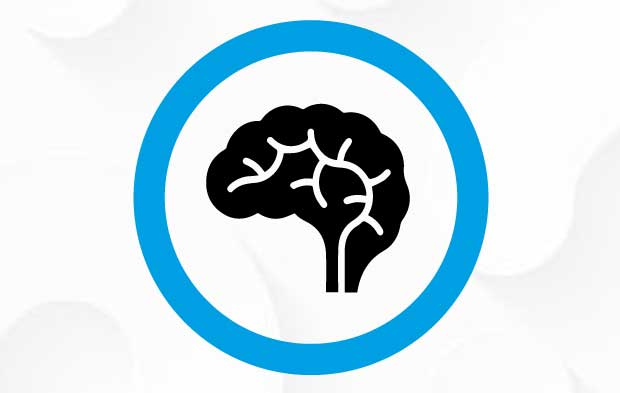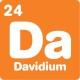# What's new## Checking the clock brainteaser

By

Terry is inspecting a huge clock. It’s 2:12 and they just finished examining the big hand. They need to check the little hand next, but they want it pointing in exactly the same direction that the big hand is pointing now. How long will they need to wait until the little hand is pointing that direction?At 2:12, the big hand is two-fifths of the way between the 2 and the 3. The little hand will be pointing the same direction, two-fifths of an hour after two.
One fifth of an hour is 12 minutes, so the big hand will be pointing in the right direction at 2:24, which is in 12 minutes!

For more brainteasers and puzzles for kids, subscribe to Double Helix magazine!Categories:

## Similar posts

1.Brainteaser. At 2:12, the big hand is ONE-fifth of the way between the 2 and the 3, not two-fifths. So it points to 11 minutes past an hour. There fore to get the small hand pointing to 3:11 one waits 59 minutes.

1.Hi Tony,
The big hand counts minutes. It pointed at the 2 at 2:10, and it will point at the 3 at 2:15, so at 1:12 it’s 2/5 of the way between the two.

2.Let x be hours as indicated by the hour hand in which there are 12 hours in a full cycle. The minutes hand is 12 minutes into a cycle of 60 minutes. By comparison

X/12 = 12/60
X=144/60
X= 2 + 24/60

Time needs to be 2:24 which is in 12min time

3.Hi. The big hand pointed at 2 at 2:00, i.e. pointed at 10 minutes past the hour. 12 minute later, at 2:12 the big hand has moved to point to 11 minutes past the hour. We want the small hand to point to 11 mins past the hour, where the big hand is at 2:12. As the small hand now points to 12 past, we must wait 59 minutes before it reaches 11 past..

4.Brenton, The problem states “They need to check the little hand next, but they want it pointing in exactly the same direction that the big hand is pointing now.” Your answer would be correct if the problem said “They need to check the BIG hand next, but they want it pointing in exactly the same direction that the LITTLE hand is pointing now. Cheers

5.Hi Folks. On my big clock the big hand is the hour hand, and the little hand is the minute hand, unlike most clocks. Sorry for the confusion!